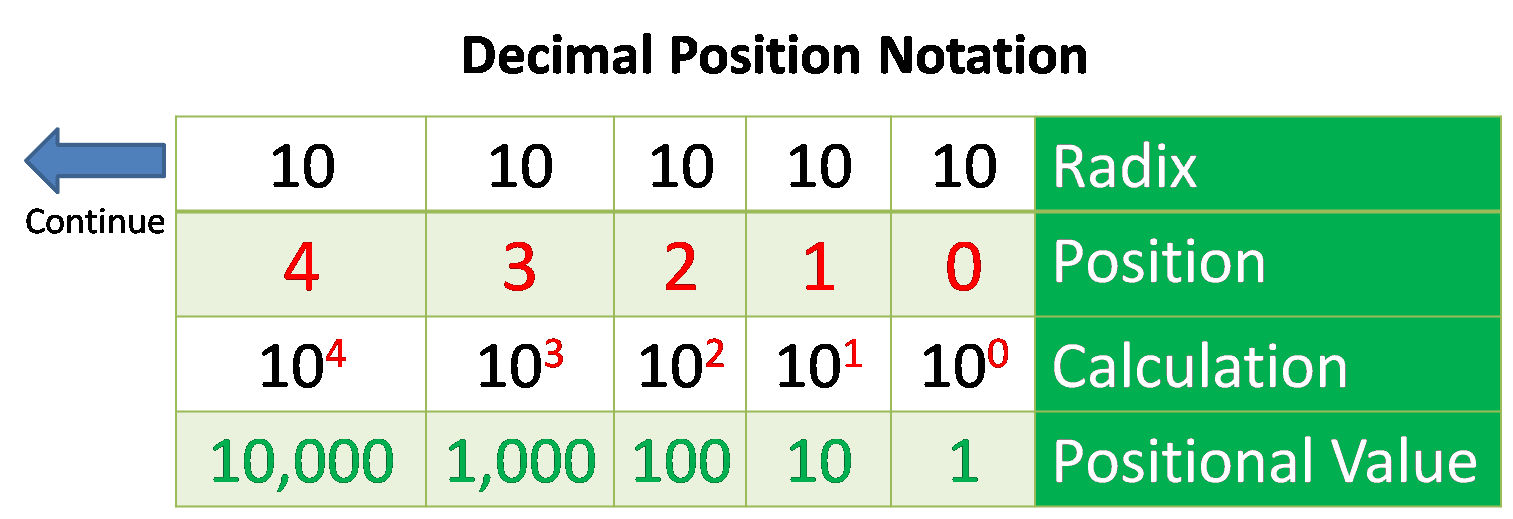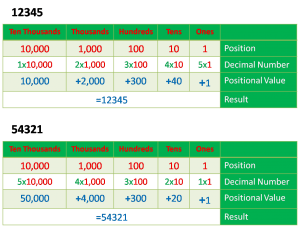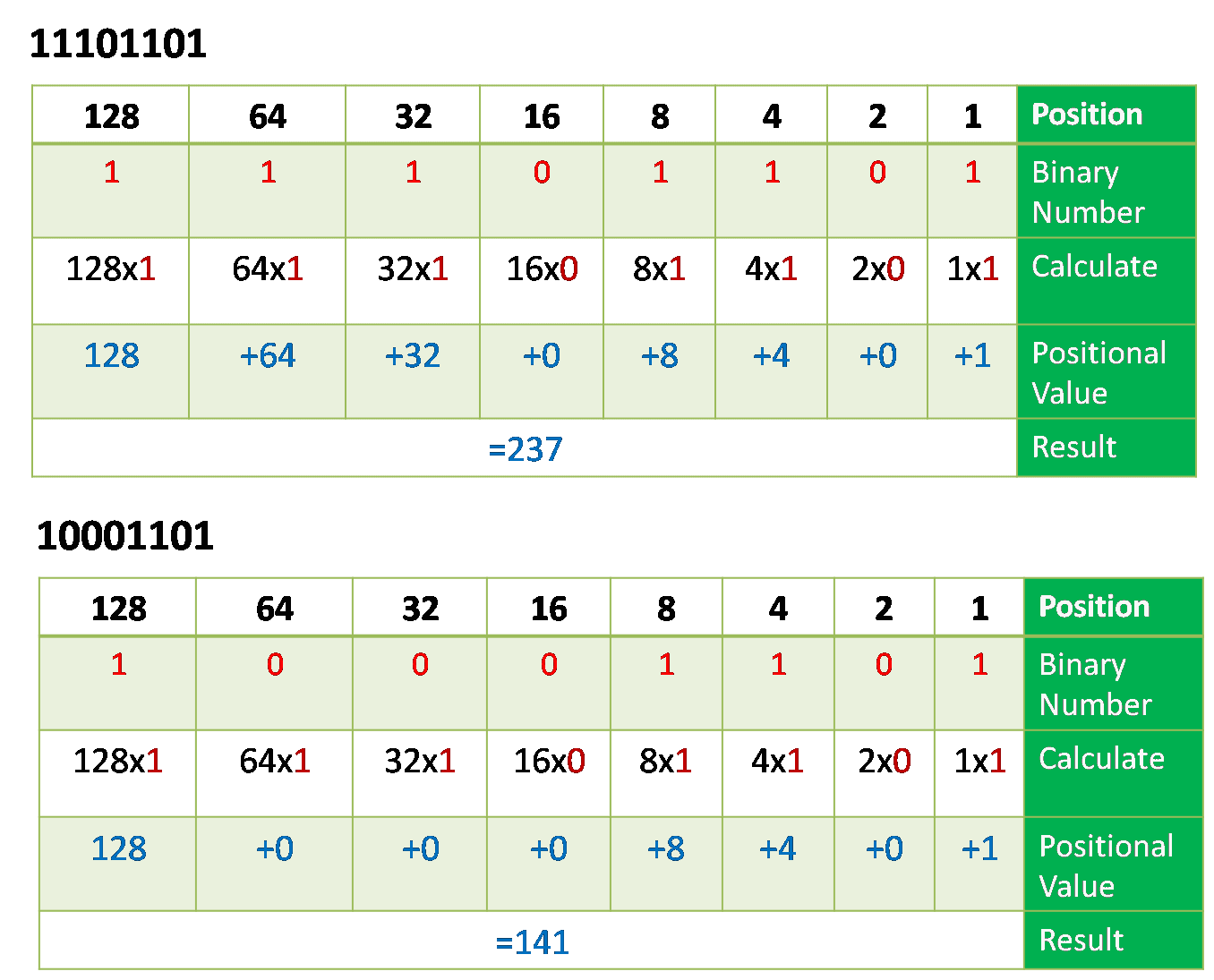# Positional Number System and Examples

In a positional number system, each symbol represents different value depending on the position they occupy in a number, and each system has a value that relates to the number directly next to it. The total value of a positional number is the total of the resultant value of all positions. The decimal number system is known as a positional number system because the value of the number depends on the position of the digits. For example, the number 12345 has a very different value than the number 54321, although the same digits are used in both numbers. The position in which the digit appears affects the value of that digit.

Contents

In a positional number system, the value of each digit determined by which place it appears in the full number. The lowest place value is the rightmost position, and each successive position to the left has a higher place value. So, the rightmost position represents the “ones” column, the next position represents the “tens” column, the next position represents “hundreds” and the next position represents the “thousand” and then ten thousand etc. Therefore, the number 54321 represents 5x ten thousand, 4x one thousand 3x hundred, 2 tens and 1 x one, whereas the number 12345 represents 1x ten thousand 2 x one thousand 3 hundred, 4 x tens and 5 ones.  The binary, octal and hexadecimal all are the examples of a Positional Number System.

## Decimal Positional Notation

The decimal positional notation system work as described in the table in the figure below. To use the positional number system, match a given number to its positional value. The arrow at the left side shows that the table can be expanded according to the position and can calculate.Term Radix is used to describe the number of digits utilized in positional number system or its identifies the positional number system base.  The decimal notation system based on 10, therefore the radix is 10.

### Position in

It shows the position of the decimal number. The position is starting from right to left. So 0 is the 1st position 1 is the 2nd position, 2 is the 3rd position and so on. The numbers also represent the exponential value that will be used to calculate the positional value of the 4th row.

### Calculate

The 3rd row calculates the positional value by taking the radix and raising it by the exponential value of its position. Important is that n0 is always = 1.

### Position Value

The first row shows the number base or radix. So the value listed, from left to right, represents units of thousands, hundreds, tens, and ones.

Example – 12345 and 54321## Binary Positional Notation

The binary positional notation operates also similar to the decimal number system as shown in the figure below. It has also a Radix, Position, which is from right to left; Calculation and positional value as describe for decimal. The Radix for Binary is 2. The example is also shown belowExamples## Converting Between Decimal and Binary

Conversion of binary to a decimal number (base-2 to base-10) numbers and back is an important concept to understand the binary numbering system. Binary number system forms the basis for all computers, digital systems, programming languages and also important to understand IP address and IP address subnetting.

### Binary to Decimal Conversion

For understanding IP addressing and subnetting, the network technician must understand how to convert from binary to decimal and back. There are several ways to convert from binary to decimal. Here we will understand conversion from binary to decimal and then from decimal to binary.

### Binary to Decimal Using Positional Notation

Positional notation is one of the most used methods for conversion between binary and decimal. So, if we have a binary number 110001102  and want to convert this number to decimal base 10 system using the positional system notation.

The figure below illustrates the conversion of the binary to decimal, write down the positional value from right to left and right down the binary number in the next row below the positional values. Next, multiply the binary numbers in the second row with its corresponding positional value in the first row and add them up to get the decimal number which is 199.The positional value is going double from right to left. For example from right to left first positional value is 1, the next position value is 2, which is double of 1 (the value of the first position), the third place value is 4 which is also double of the 2nd position value. We can write positional value by this method according to the digits of binary.

The next binary value is 10111100 So,  write the positional value according to the number of the digits in the binary value. And then write the binary numbers below the positional values and map them up. Now If the digit is a 1, write its corresponding positional value below the line, under the digit. If the digit is a 0, write a 0 below the line, under the digit and, add up the numbers written below the line. This is the decimal equivalent of the binary number. See figure the figure below.Now we understand the basic conversion from binary to decimal, so we can try to convert a binary IPv4 address to its dotted-decimal equal. First, divide 32 bits of the IPv4 address into four 8-bit octets. Then calculate the binary positional value to the first octet binary number and calculate all others so.

For example, consider that 11110110.10111111.11101011.11011011 is the binary IPv4 address. You need to convert the binary address to decimal, simply start from the first octet as shown in Figure below. Enter the 8-bit binary number under the positional value of row 1 and then calculate to produce the decimal number for the first octet of the dotted decimal notation. Next, convert the remaining octets. And write down the full IP address in dotted decimal notation.### Decimal to Binary Conversion

Decimal to binary conversion is also important to understand, we can have to convert a dotted decimal IPv4 address to binary. For converting from decimal to binary, we can also use positional value table. The figure below illustrates the decimal to the binary conversion process. For example, we want to convert figure 246 into binary. The steps for converting from decimal to binary are the following:Step-1

Write down the positional value from left to write as shown in the figure below. If the decimal number (242 in our case) is equal to or greater than the most significant bit (128). If yes, add a binary 1 below the 128 positional value and subtract 128 from the decimal number (242 -128 =114  in our case). If no, then enter binary 0 below the 128 positional value.

Step-2

Compare if the remainder (114) is equal to or greater than the next-most-significant bit (64). If yes, add a binary 1 below the 64 positional value and subtract the positional value 64 from the decimal number (114 -64 =50  in our case). If no, then enter binary 0 below the 64 positional value.

Step-3

Compare if the remainder (50) is equal to or greater than the next-most-significant bit (32). If yes, add a binary 1 below the 32 positional value and subtract positional value 32 from the decimal number (50 -32 =18  in our case). If no, then enter binary 0 below the 64 positional value.

Step-4

Compare if the remainder (18) is equal to or greater than the next-most-significant bit (16). If yes, add a binary 1 below the 16 positional value and subtract positional value 16 from the decimal number (18 -16 =2  in our case). If no, then enter binary 0 below the 16 positional value.

Step-5

Compare if the reminder (2) with the next -most significant bit, you can see that the remainder is less than the significant value (80), so, add a binary 0 in the 8 positional value, and step forward to next positional value which is (4), the reminder is also less than this positional value, so, add 0 below this position value and step forward to next value. The next value is positional value is (2), So, Subtract the reminder 2 from the positional value (2-2=0), put the 0 in the last position.